Next: 13.3.4 The Convolution Extension Up: 13.3 Convolutions Previous: 13.3.2.2 Separable Filters   Contents

### 13.3.3 Convolutions Using the Accumulation Buffer

The convolution operation may be implemented by building the output image in the accumulation buffer. For each kernel entry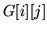, translate the input image by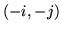from its original position and then accumulate the translated image using the command glAccumGL_ ACCUM, G[i][j](GL_ ACCUM, G[i][j]). This translation can be performed by glCopyPixels() but an application may be able to more efficiently redraw the image shifted using glViewport().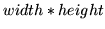translations and accumulations must be performed. Skip clearing the accumulation buffer by using GL_ LOAD instead of GL_ ACCUM for the first accumulation.

Here is an example of using the accumulation buffer to convolve using a Sobel filter, commonly used to do edge detection. This filter is used to find horizontal edges: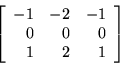Since the accumulation buffer can only store values in the range (-1..1), first modify the kernel such that at any point in the computation the values do not exceed this range: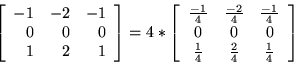The operations needed to apply the filter are:
1. Draw the input image.
2. glAccumGL_ LOAD, 1/4(GL_ LOAD, 1/4)
3. Translate the input image left by one pixel.
4. glAccumGL_ ACCUM, 2/4(GL_ ACCUM, 2/4)
5. Translate the input image left by one pixel.
6. glAccumGL_ ACCUM, 1/4(GL_ ACCUM, 1/4)
7. Translate the input image right by two pixels and down by two pixels.
8. glAccumGL_ ACCUM, -1/4(GL_ ACCUM, -1/4)
9. Translate the input image left by one pixel.
10. glAccumGL_ ACCUM, -2/4(GL_ ACCUM, -2/4)
11. Translate the input image left by one pixel.
12. glAccumGL_ ACCUM, -1/4(GL_ ACCUM, -1/4)
13. Return the results to the framebuffer (glAccumGL_ RETURN, 4(GL_ RETURN, 4)).
In this example, each pixel in the output image is the combination of pixels in the 3 by 3 pixel square whose lower left corner is at the output pixel. At each step, the image is shifted so that the pixel that would have been under the kernel element with the value used is under the lower left corner. As an optimization, ignore locations where the kernel is equal to zero.

A general algorithm for the 2D convolution operation is:

Draw the input image
for (j = 0; j < height; j++) {
for (i = 0; i < width; i++) {
glAccum(GL_ACCUM, G[i][j]*scale);
Move or redraw the input image to the left by 1 pixel
}
Move or redraw the input image to the right by width pixels
Move or redraw the input image down by 1 pixel
}
glAccum(GL_RETURN, 1/scale);

scale is a value chosen to ensure that the intermediate results cannot go outside a certain range. In the Sobel filter example, scale = 4. Assuming the input values are in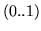, scale can be naively computed using the following algorithm:
float minPossible = 0, maxPossible = 1;
for (j = 0; j < height; j++) {
for (i = 0; i < width; i++) {
if (G[i][j] < 0) {
minPossible += G[i][j];
} else {
maxPossible += G[i][j];
}
}
}
scale = 1.0 / ((-minPossible > maxPossible) ?
-minPossible : maxPossible);

Since the accumulation buffer has limited precision, more accurate results can be obtained by changing the order of the computation and computing scale accordingly. Additionally, if values in the input image can be constrained to a smaller range, scale can be made larger, which may also give more accurate results.

For separable kernels, convolution can be implemented using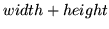image translations and accumulations. A general algorithm is:

Draw the input image
for (i = 0; i < width; i++) {
glAccum(GL_ACCUM, Grow[i] * rowScale);
Move or redraw the input image to the left 1 pixel
}
glAccum(GL_RETURN, 1 / rowScale);
for (j = 0; j < height; j++) {
glAccum(GL_ACCUM, Gcol[j] * colScale);
Move or redraw the framebuffer image down by 1 pixel
}
glAccum(GL_RETURN, 1 / colScale);

In this example, it is assumed that scales for the row and column filters have been determined in a similar fashion to the general two-dimensional filter, such that the accumulation buffer values will never go out of range.Next: 13.3.4 The Convolution Extension Up: 13.3 Convolutions Previous: 13.3.2.2 Separable Filters   Contents
2001-01-10